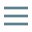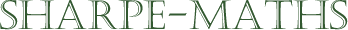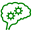General puzzle page
•A boy has twice as much money as his friend. If they have 84p together, how much does each have?
•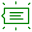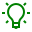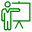•Along a certain street the lights are placed $30m$ apart. If there are $10$ lights, how far is it from the first to the last?

••$2$ ice-creams are eaten by $2$ children in $2$ minutes. How long will it take $5$ children to eat $5$ ice-creams?

••An electric train is traveling due north. If the wind is blowing from the north west, in which direction is the smoke blowing?

••Two farmers are taking their cows to market. On the way, one says to the other, "If you give me one of your cows, we would have the same number of cows." The other farmer replies, "True, but if you give me one of your cows, I will have twice as m any as you.". How many cows did the two farmers each have?
••A window frame in Salt's Mill, just outside Bradford in Yorkshire, consists of two equal semi-circles and a circle inside a large semi-circle with each touching the other three as shown. The width of the frame is $4m$.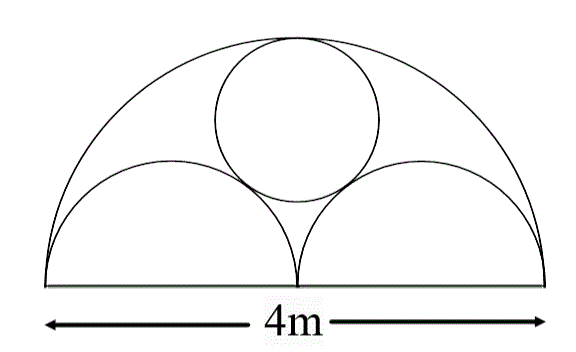What is the radius of the circle in metres?

••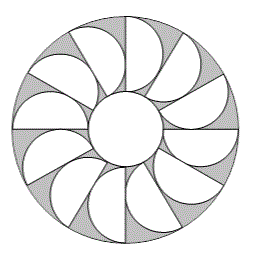The diagram shows an annulus, which is the region between two circles with the same centre. Twelve equal touching semi-circles are placed inside the annulus. The diameters of the semi-circles lie along the diameters of the outer circle.

What fraction of the annulus is shaded?

••Three squares are drawn on the outside of a right angled triangle, whose shorter sides have lengths $a$ and $2a$. The whole figure is surrounded by a rectangle, as shown.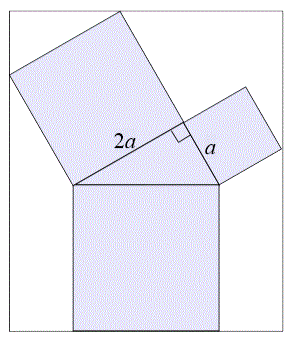What is the ratio of the area of the shaded region to the area of the outer rectangle?

•